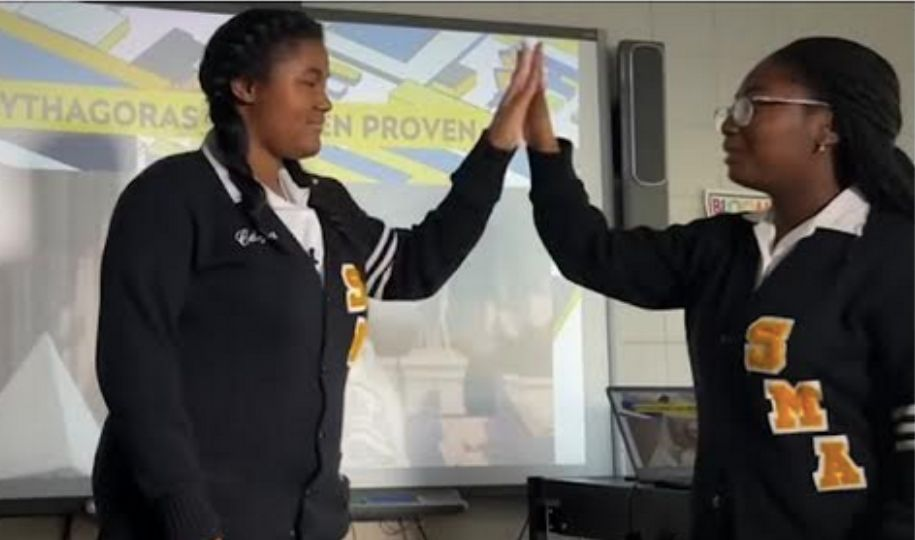# New Orleans Teens Say They Have Solved 2,000-Year-Old Mathematical TheoremCalcea Johnson (L) and Ne’Kiya Jackson (R). (Photo: Screenshot, WWLTV)

Calcea Johnson and Ne’Kiya Jackson are two New Orleans high school students who say they have solved a 2,000-year-old mathematical theorem. The pair recently presented their findings on the Pythagorean Theorem to a group of professional mathematicians.

Johnson and Jackson are both 17-year-old high school seniors set to graduate this spring.

Pythagorean theorem is a geometric theorem that the sum of the squares on the legs of a right triangle is equal to the square on the hypotenuse (the side opposite the right angle)—or, in familiar algebraic notation, a2 + b2 = c2.

Academics have long thought it was impossible to solve the theorem using trigonometry.

Johnson and Jackson attend St Mary’s Academy, an all-girls school in the city’s Plum Orchard neighborhood. They gave a presentation on March 18 of their findings at the American Mathematical Society south-eastern chapter’s semi-annual meeting in Georgia, The Guardian reported. The society has used the teens to submit their findings to an industry journal.

In the abstract of Johnson and Jackson’s mathematical society presentation, they prove the theorem using trigonometry, which has never been done. It has been proved extensively by other means, Insider reported.

Elisha Loomis’ book The Pythagorean Proposition contains the largest known collection of proofs for the theorem. The book “flatly states that ‘there are no trigonometric proofs because all the fundamental formulae of trigonometry are themselves based upon the truth of the Pythagorean theorem’.”

But the two teens said, “that isn’t quite true.”

“We present a new proof of Pythagoras’s Theorem, which is based on a fundamental result in trigonometry – the Law of Sines – and we show that the proof is independent of the Pythagorean trig identity sin2x+cos2x=1,” they state in their abstract. They prove the theorem using trigonometry and without using circular reasoning.

Calcea Johnson (L) and Ne’Kiya Jackson (R). (Photo: Screenshot, WWLTV, https://www.youtube.com/watch?v=Ka1k4i1ueNU&embeds_euri=https%3A%2F%2Fwww.theguardian.com%2F&feature=emb_imp_woyt)